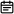# 938. 二叉搜索树的范围和2019-06-06

## 题目链接

https://leetcode-cn.com/problems/range-sum-of-bst/

## 题目描述

``````输入：root = [10,5,15,3,7,null,18], L = 7, R = 15

``````输入：root = [10,5,15,3,7,13,18,1,null,6], L = 6, R = 10

## 解题方案

### 思路

• 标签：深度优先遍历
• 题意：这个题字面含义很难理解，本意就是求出所有 `X >= L``X <= R` 的值的和
• 递归终止条件：

• 当前节点为null时返回0
• 当前节点 `X < L` 时则返回右子树之和
• 当前节点 `X > R` 时则返回左子树之和
• 当前节点 `X >= L``X <= R` 时则返回：当前节点值 + 左子树之和 + 右子树之和
• 注意点：通过判断X的大小能够避免遍历全部树的节点，比如下方的动图中，3这个值就没有必要遍历

### 代码

``````/**
* Definition for a binary tree node.
* public class TreeNode {
*     int val;
*     TreeNode left;
*     TreeNode right;
*     TreeNode(int x) { val = x; }
* }
*/
class Solution {
public int rangeSumBST(TreeNode root, int L, int R) {
if (root == null) {
return 0;
}
if (root.val < L) {
return rangeSumBST(root.right, L, R);
}
if (root.val > R) {
return rangeSumBST(root.left, L, R);
}
return root.val + rangeSumBST(root.left, L, R) + rangeSumBST(root.right, L, R);
}
}``````

### 画解